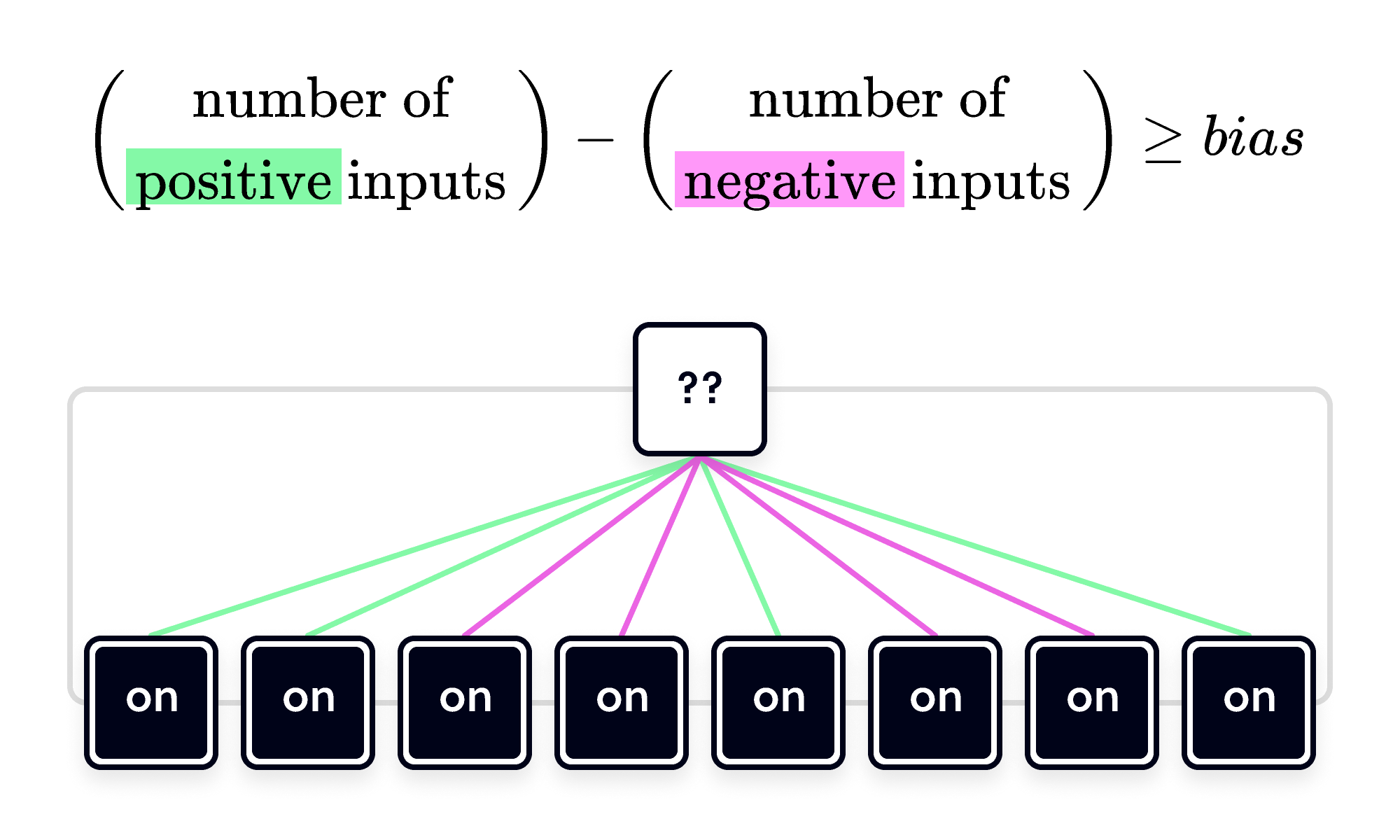Back

## All the Way to the Top

Artificial neural networks (ANNs) can identify patterns in data, like whether or not an animal is in a photo or cancerous growth is in an MRI image. The basic unit of an ANN is the artificial neuron, inspired by simplified mathematical models of the neurons in animal brains.

Just as biological neurons send electrical impulses to other neurons and change their own state based on the pulses they receive, an artificial neuron can be active $(\blacksquare)$ or inactive $(\square)$ based on the information it receives from its inputs.

The single neuron below has $3$ inputs, which can be on oroff. Clicking on an input will change its state. Can you find the input pattern that activates the neuron?

The inputs with green edges drive the neuron toward its active state $(\blacksquare).$ When the pink input is on, it has the opposite effect; it inhibits the neuron. The neuron's output state is determined by summing the inputs: each green edge contributes $+1$ and pink one contributes $-1.$ (Only the inputs that are on are counted; inputs switched off have no effect.)

When the sum of the inputs equals or exceeds a particular value, called its bias, the neuron flips from $\square$ to $\blacksquare.$ The higher the bias, the greater the input needed to flip the neuron. The bias of the neuron above is $+2.$ It takes $2$ positive inputs to flip the neuron to $\blacksquare.$

A single rule governs whether a neuron is activated by its inputs:This simple rule can give rise to complex behavior when multiple neurons are connected in a neural network. Typically, neurons in a network transform the inputs across a series of "layers."

In the network below, neurons are organized into three layers.

The inputs make up the bottom layer, the middle layer has two neurons that can be activated by the inputs, and the top layer (the output) consists of one neuron. The inputs to the top neuron are the two neurons in the middle layer.

When all three inputs are on, only the right neuron in the middle layer is active, which isn't sufficient to activate the output neuron at the top. The biases of all three neurons are fixed at $+2.$ Can you figure out how to activate the output neuron?

The solution is to switch off the negative input. This increases the input to the left neuron in the middle layer. Both are activated, which in turn increases the input to the output neuron.

The input combination (on, off, on) is the only one that activates the output neuron. If only one input is on — for example, $($on, off, off$)$ or $($off, off, on$)$ — the input to each neuron in the middle layer doesn't equal or exceed the bias $+2,$ so they remain inactive.

Since there are $2$ states for each of the three inputs, there are $2\times 2\times 2=8$ possible input combinations, and $7$ combinations fail to activate the output neuron.

# Today's Challenge

In the tree-shaped neural network below, there are $5$ inputs and $10$ neurons, which all have a bias of $+2.$

The green edges are $+1$ and the pink edges are $-1.$

How many of the $32$ possible input combinations activate the output neuron?We are sunsetting our community features by July 2, 2021. Learn more here.
×

Problem Loading...

Note Loading...

Set Loading...